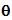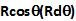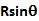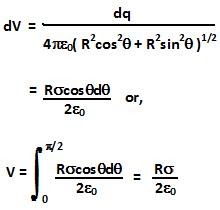## Sunday, May 9, 2010

### Irodov Problem 3.32

The hollow hemispherical bowl can be thought of being composed several infinitesimally thin rings. Consider an infinitesimally thin ring (shaded as red in the figure) that is at an elevation angleand its thickness subtends an angle. The thickenss of this ring will beand its radius will be. The total surface area of this thin ring will hence be. The total charge contained in this ring will hence be,

Now in problem 3.9 we have already computed the electric field due to a ring at points along its axis. Since, the center of the hemisphere is at a distancefrom this infinitesimally thin ring
(see in the figure), using this result we can compute the electric field due to this inifinitesimally thin ring as,

Isnt't it interesting that the electric field strength at the center does not depend on the radius R of the bowl? The net electric field can be determined as,

Similary, we can determine the potential at the center of the infnitesimally thin ring by using the result derived in Problem 3.30 Eqn 1 and use it to determine the potential at the center of the hemisphere as,1.2.